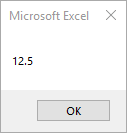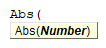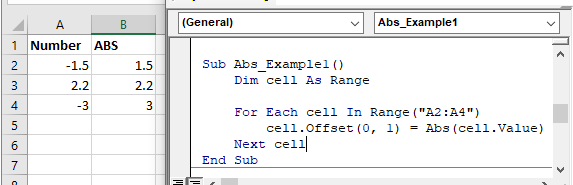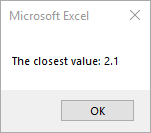# VBA Abs Function

## Abs Description

Returns the absolute value of a number.

## Simple Abs Examples

``````Sub Abs_Example()
MsgBox Abs(-12.5)
End Sub
``````

This code will return 12.5## Abs Syntax

In the VBA Editor, you can type  “Abs(” to see the syntax for the Abs Function:The Abs function contains an argument:

Number: A numeric value.

## Examples of Excel VBA Abs Function

you can reference a cell containing a date:``````Sub Abs_Example1()
Dim cell As Range

For Each cell In Range("A2:A4")
cell.Offset(0, 1) = Abs(cell.Value)
Next cell
End Sub``````

The result will be as following.(please see B2:B4)The following 2 examples both will return 12.

``MsgBox Abs(-12)``
``MsgBox Abs(12)``

To find a number closest to 2 when a number array (1.5, 3.1, 2.1, 2.2, 1.8) is given, you can use the following code.

``````Sub Abs_Example2()
Dim Numbers
Dim item
Dim closestValue As Double
Dim diff As Double
Dim minDiff As Double
minDiff = 100

Numbers = Array(1.5, 3.1, 2.1, 2.2, 1.8)

For Each item In Numbers
diff = Abs(item - 2)
If diff < minDiff Then
minDiff = diff
closestValue = item
End If
Next item

MsgBox "The closest value: " & closestValue
End Sub``````

The result will be 2.1 as following.Stop searching for VBA code online. Learn more about AutoMacro - A VBA Code Builder that allows beginners to code procedures from scratch with minimal coding knowledge and with many time-saving features for all users!